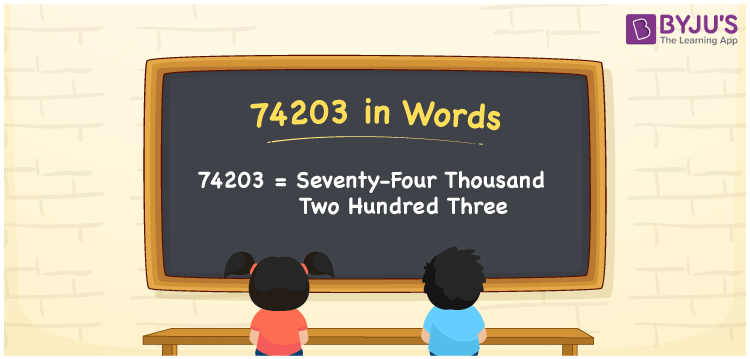# 74203 in Words

74203 in words is written as Seventy-four two hundred three. In both the International System of Numerals and the Indian System of Numerals, 74203 is written as Seventy-four two hundred three. The number 74203 is a Cardinal Number as it denotes some quantity. For example, “that bike costs 74203 rupees”.

 74203 in Words Seventy-four two hundred three Seventy-four two hundred three in Number 74203

## 74203 in English Words

74203 in English words is read as “Seventy-four two hundred three”.## How to Write 74203 in Words?

To write 74203 in words, we shall use the place value chart. In the place value chart, put 7 in the ten thousands, 4 in the thousands, 2 in the hundreds, 0 in the tens and 3 in the ones. Let us make a place value chart to write the number 74203 in words.

 Ten Thousands Thousands Hundreds Tens Ones 7 4 2 0 3

Thus, we can write the expanded form as

7 × Ten Thousand + 4 × Thousand + 2 × Hundred + 0 × Ten + 3 × One

= 7 × 10000 + 4 × 1000 + 2 × 100 + 0 × 10 + 3 × 1

= 70000 + 4000 + 200 + 0 + 3

= 74203

= Seventy-four two hundred three.

74203 is a natural number, the successor of 74202 and the predecessor of 74204.

74203 in words – Seventy-four two hundred three

• Is 74203 an odd number? – Yes
• Is 74203 an even number? – No
• Is 74203 a perfect square number? – No
• Is 74203 a perfect cube number? – No
• Is 74203 a prime number? – Yes
• Is 74203 a composite number? – No

## Frequently Asked Questions on 74203 in Words

Q1

### How to write 74203 in words?

74203 in words is written as Seventy-four two hundred three.
Q2

### How to write 74203 in the International and Indian System of Numerals?

In both, the system of numerals, 74203 in words, is written as Seventy-four two hundred three.
Q3

### How to write 74203 in a place value chart?

In the place value chart, write 7 in the ten thousands, 4 in the thousands, 2 in the hundreds, 0 in the tens and 3 in the ones, respectively.